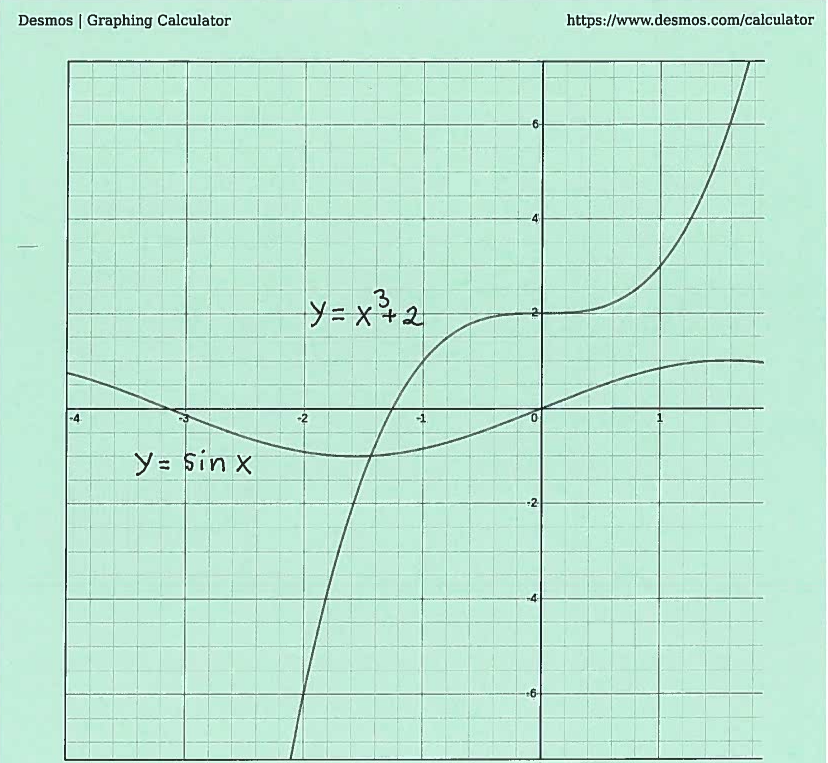SOLUTION 7: We are given the equation $$x^3+2= \sin{x} \ \ \ \ \longrightarrow \ \ \ \ x^3+2-\sin{x}=0$$ Let function $$f(x)=x^3+2-\sin{x} \ \ \ \ and \ choose \ \ \ \ m=0$$ This function is continuous for all values of $x$ since it is the DIFFERENCE of continuous functions; it is well-known that $\sin{x}$ is continuous for all values of $x$ and $x^3+2$ is continuous for all values of $x$ since it is a polynomial. To establish an appropriate interval consider the graph of this function. (Please note that the graph of the function is not necessary for a valid proof, but the graph will help us understand how to use the Intermediate Value Theorem. On many subsequent problems, we will solve the problem without using the "luxury" of a graph.)Note that $$f(0)= (0)^3+2-\sin{(0)} =2>0 \ \ \ \ and \ \ \ \ f(-\pi)= (-\pi)^3+2-\sin{(-\pi)}= -\pi^3+2-0=2-\pi^3 \approx -29<0$$
so that $$f(-\pi) \approx -29 < m <2=f(0)$$
i.e., $m=0$ is between $f(-\pi)$ and $f(0)$. Now choose the interval to be $\ [-\pi, 0]$.

The assumptions of the Intermediate Value Theorem have now been met, so we can conclude that there is some number $c$ in the interval $[-\pi, 0]$ which satisfies $$f(c)=m$$ i.e., $$c^3+2-\sin{c}=0$$ and the equation is solvable.